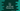# C++ program to find the nth odd Fibonacci number## C++ program to find the nth odd Fibonacci number:

In this post, we will learn how to find the nth odd Fibonacci number in C++. The Fibonacci number sequence, also known as Fibonacci series is a series of numbers that starts from 0 and 1 and each value is sum of two preceding ones.

For example, below is the starting numbers of Fibonacci series:

``0, 1, 1, 2, 3, 5, 8, 13, 21, 34, 55, 89, 144, 233, 377, 610, 987, 1597, 2584, 4181, 6765, 10946, 17711.....``

Here, the first odd Fibonacci number is 1, second is 1, third one is 3 etc. So, we will take the value of n and find out the nth odd fibonacci number from the fibonacci series.

### C++ program to find the nth odd Fibonacci number:

Below program is used to find the nth odd Fibonacci :

``````#include <iostream>
using namespace std;

int getNthOddFibonacci(int n)
{
int currentOdd = 1;
int prev = 0;
int curr = 1;
int temp;
int oddNumber = -1;

bool oddFound = false;

if(n == 1){
return 1;
}

while(!oddFound){
temp = curr;
curr = curr + prev;
prev = temp;

if(curr % 2 != 0){
currentOdd++;
if(currentOdd == n){
oddNumber = curr;
oddFound = true;
}
}
}

return oddNumber;
}

int main()
{
int n;

cout << "Enter the value of n : " << endl;
cin >> n;

cout << "nth odd Fibonacci number is : " << getNthOddFibonacci(n) << endl;
}``````

Here,

• getNthOddFibonacci is used to get the nth odd fibonacci number.
• currentOdd is used to keep the current odd number found. This is incremented by 1 if we get any odd number in the Fibonacci series. Once it is equal to the user given value of n, it sets the value to oddNumber and mark oddFound flag as true, i.e. it will exit from the while loop.
• It returns the value of oddNumbers.

Sample output:

``````Enter the value of n :
12
nth odd Fibonacci number is : 1597``````

### Method 2: Using a for loop:

Another way to find the nth odd fibonacci number is by using a for loop. If I write down only the odd fibonacci values, then the series will look like:

``1, 1, 3, 5, 13, 21, 55, 89, 233, 377,....``

If you look closely, you will find that nth odd fibonacci number in the series is equal to (3*n + 1)/2th value in the fibonacci series. So, we can just use a for loop to find that number in the fibonacci series:

``````#include <iostream>
using namespace std;

int getNthOddFibonacci(int n)
{
int prev = 0;
int curr = 1;
int temp;

if(n == 1){
return 1;
}

for(int i = 2; i < (3*n +1)/2 ; i++){
temp = curr;
curr = curr + prev;
prev = temp;
}

return curr;
}

int main()
{
int n;

cout << "Enter the value of n : " << endl;
cin >> n;

cout << "nth odd Fibonacci number is : " << getNthOddFibonacci(n) << endl;
}``````

Here,

• The for loop runs from i = 2. Because, the first and the second numbers are 0 and 1.
• At the end of the loop, curr or current value of the Fibonacci series will hold the nth odd Fibonacci value. So, this method is returning this value.

It will give similar output as the previous example.